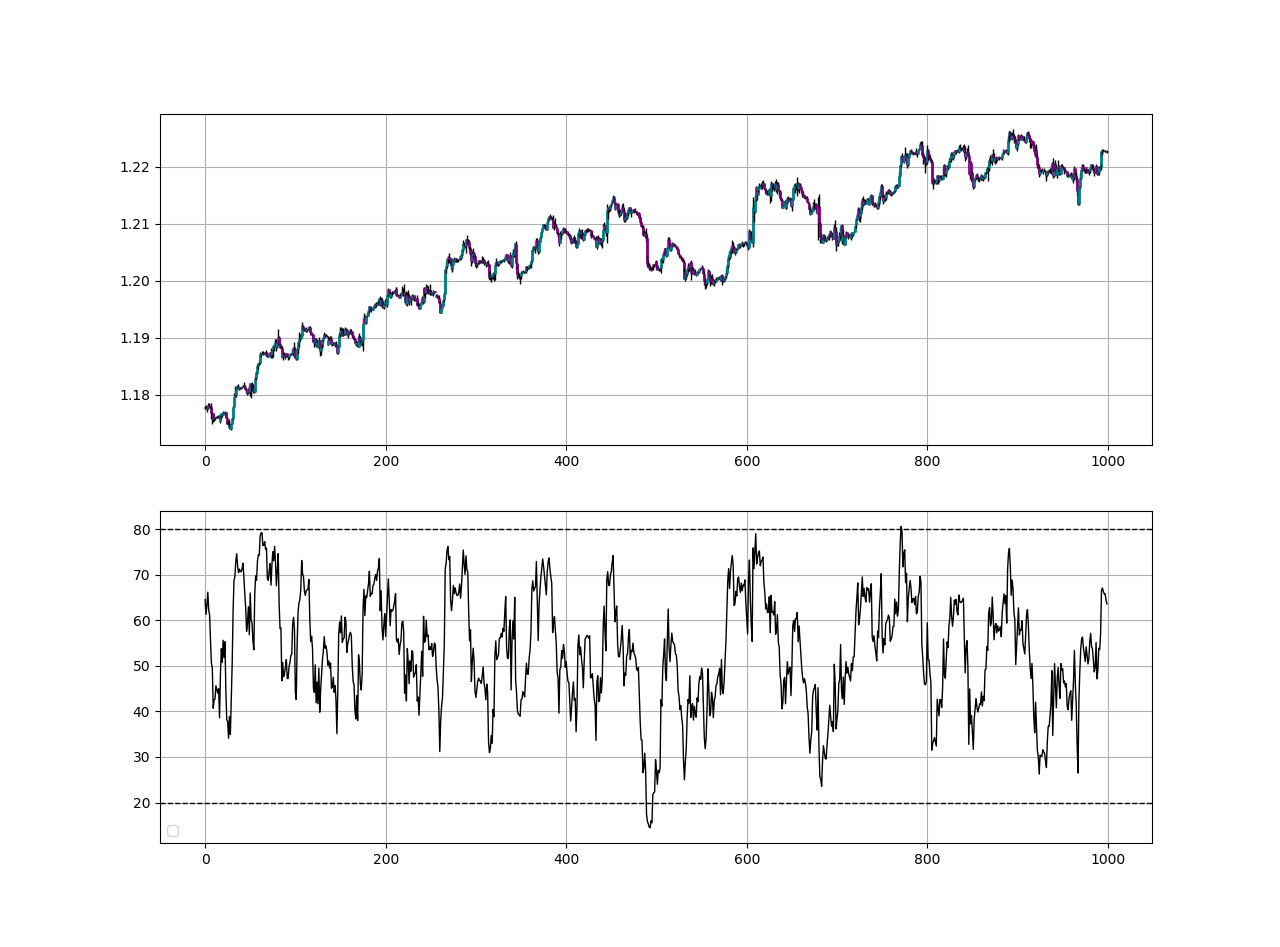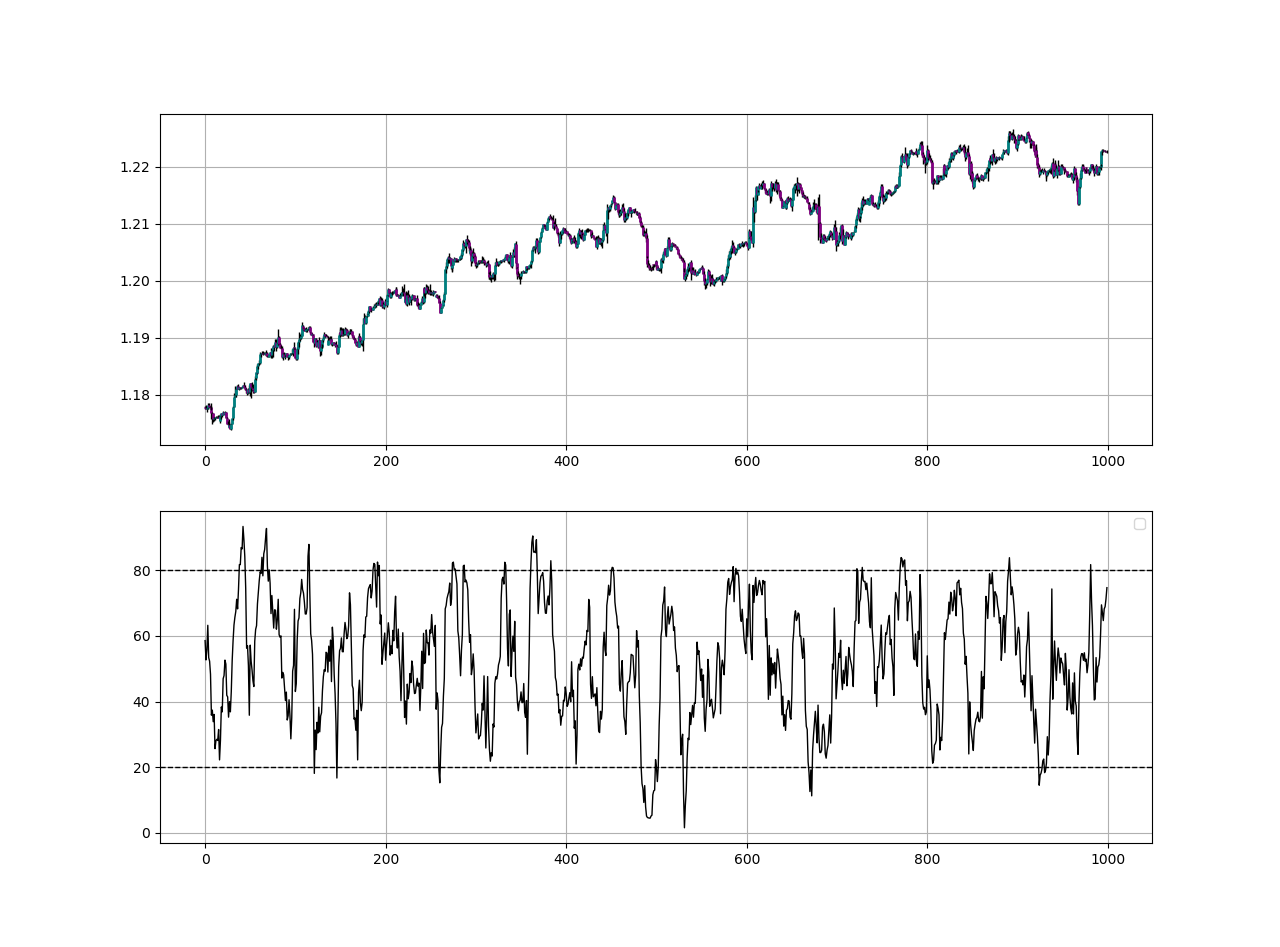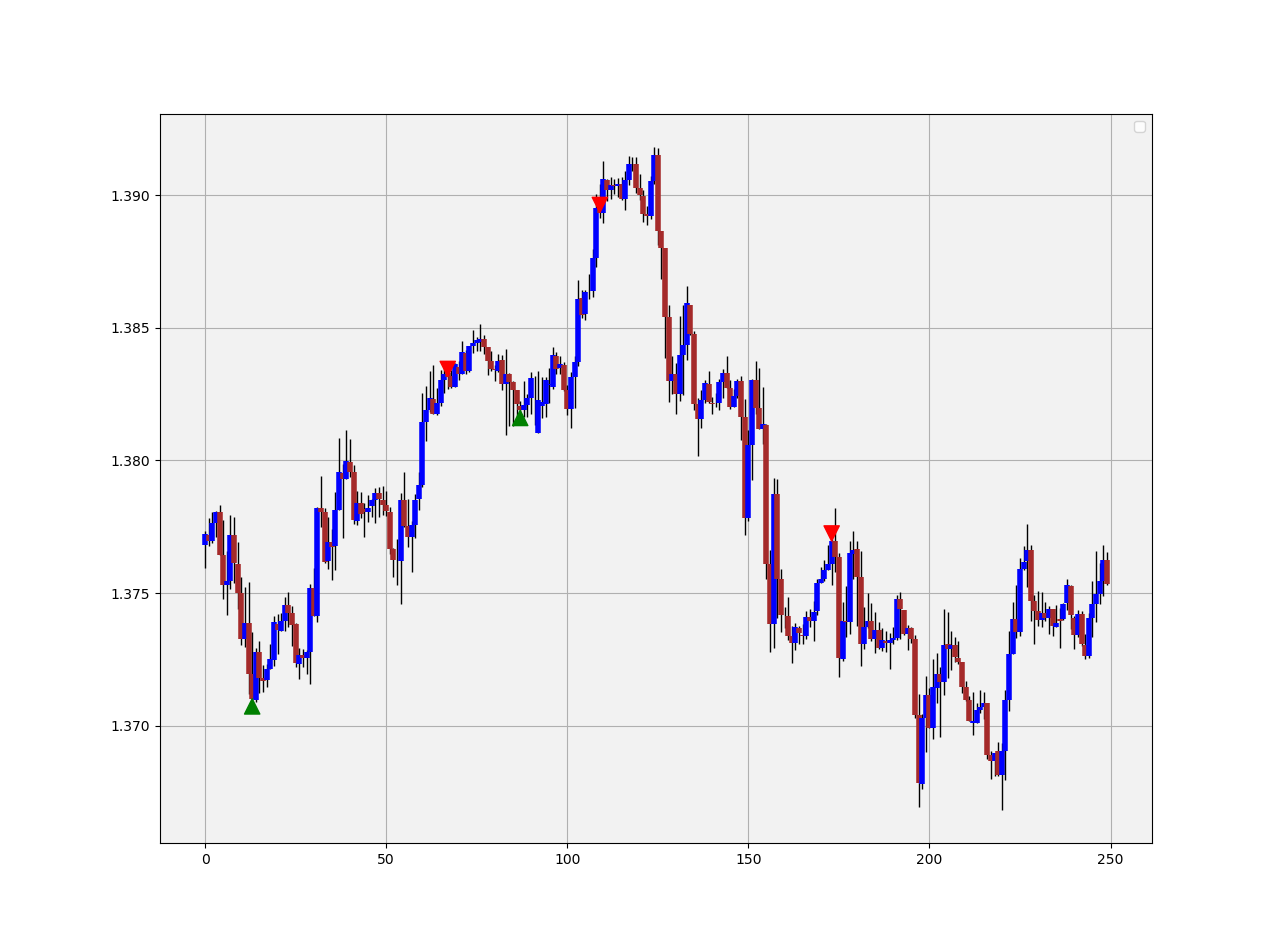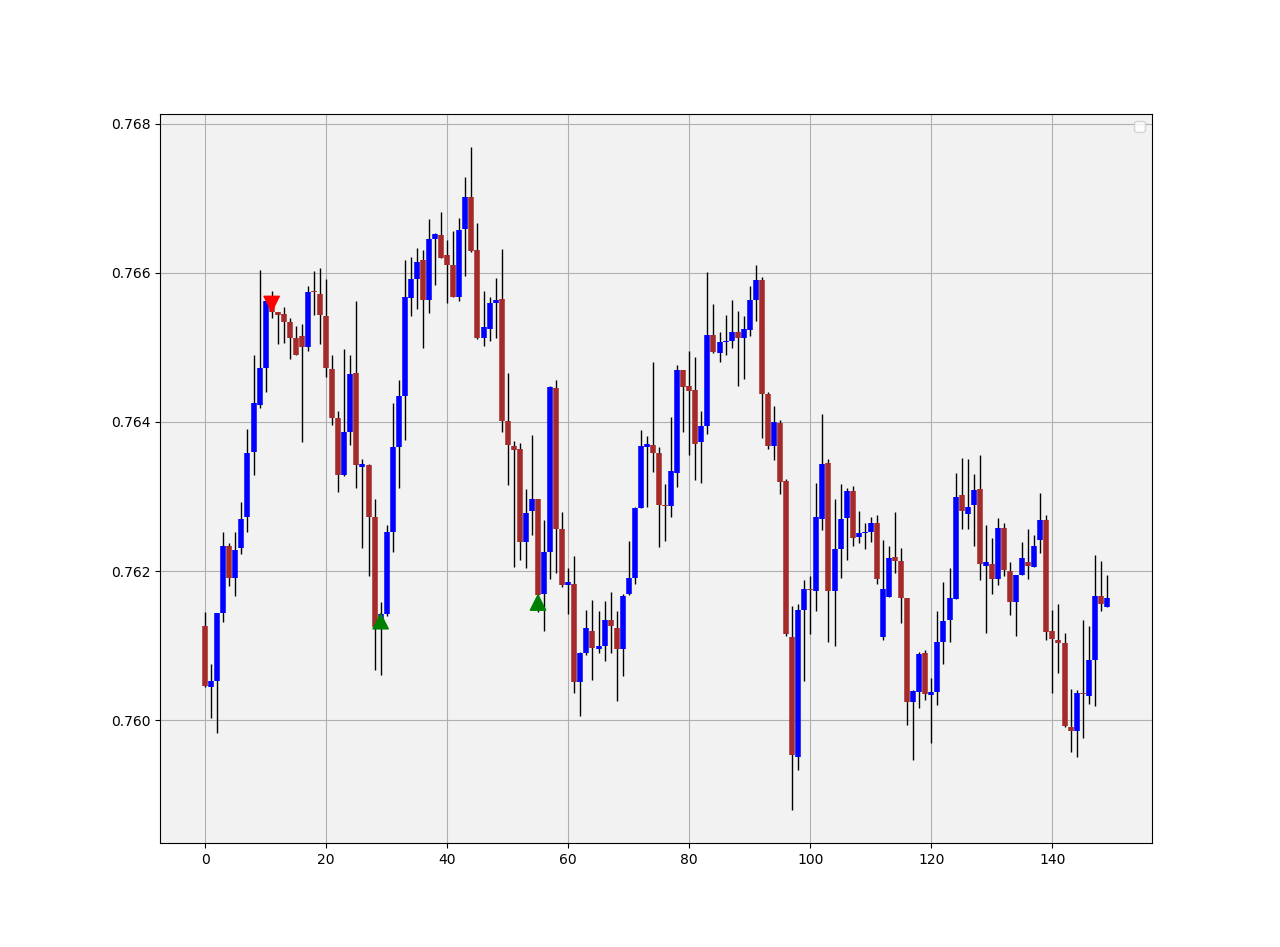# How to Apply Timing Patterns on Technical Indicators Such as the RSI in Python.Sofienne Kaabar

2 years ago | 26 min read

Timing patterns are configurations that occur regularly and may have an expected outcome with a probability of slightly more than 50%. This is defined as a predictive pattern.

Typically, we apply the rules of the patterns on the market price. However, we can also apply them on transformations of the market price such as technical indicators. In this article, we will apply the Fibonacci Timing Pattern on the values of the well-known Relative Strength Index to understand the quality of the signals generated.

If you are also interested by more technical indicators and using Python to create strategies, then my latest book may interest you:

# The Simple Relative Strength Index

The RSI is without a doubt the most famous momentum indicator out there, and this is to be expected as it has many strengths especially in ranging markets. It is also bounded between 0 and 100 which makes it easier to interpret. Also, the fact that it is famous, contributes to its potential.
This is because the more traders and portfolio managers look at the RSI, the more people will react based on its signals and this in turn can push market prices. Of course, we cannot prove this idea, but it is intuitive as one of the basis of Technical Analysis is that it is self-fulfilling.

The RSI is calculated using a rather simple way. We first start by taking price differences of one period. This means that we have to subtract every closing price from the one before it.

Then, we will calculate the smoothed average of the positive differences and divide it by the smoothed average of the negative differences. The last calculation gives us the Relative Strength which is then used in the RSI formula to be transformed into a measure between 0 and 100.EURUSD hourly values in the first panel with the 14-period Standard RSI.

To calculate the Relative Strength Index, we need an OHLC array (not a data frame). This means that we will be looking at an array of 4 columns. The function for the Relative Strength Index is therefore:

`def rsi(Data, lookback, close, where, width = 1, genre = 'Smoothed'):        # Adding a few columns    Data = adder(Data, 7)        # Calculating Differences    for i in range(len(Data)):                Data[i, where] = Data[i, close] - Data[i - width, close]         # Calculating the Up and Down absolute values    for i in range(len(Data)):                if Data[i, where] > 0:                        Data[i, where + 1] = Data[i, where]                    elif Data[i, where] < 0:                        Data[i, where + 2] = abs(Data[i, where])                # Calculating the Smoothed Moving Average on Up and Down    absolute values        if genre == 'Smoothed':                          lookback = (lookback * 2) - 1 # From exponential to smoothed      Data = ema(Data, 2, lookback, where + 1, where + 3)      Data = ema(Data, 2, lookback, where + 2, where + 4)        if genre == 'Simple':                          Data = ma(Data, lookback, where + 1, where + 3)      Data = ma(Data, lookback, where + 2, where + 4)        # Calculating the Relative Strength    Data[:, where + 5] = Data[:, where + 3] / Data[:, where + 4]        # Calculate the Relative Strength Index    Data[:, where + 6] = (100 - (100 / (1 + Data[:, where + 5])))          # Cleaning    Data = deleter(Data, where, 6)    Data = jump(Data, lookback)                        return Data`

We need to define the primal manipulation functions first in order to use the RSI’s function on OHLC data arrays.

`# The function to add a certain number of columnsdef adder(Data, times):        for i in range(1, times + 1):            z = np.zeros((len(Data), 1), dtype = float)        Data = np.append(Data, z, axis = 1)                return Data# The function to deleter a certain number of columnsdef deleter(Data, index, times):        for i in range(1, times + 1):            Data = np.delete(Data, index, axis = 1)                       return Data# The function to delete a certain number of rows from the beginningdef jump(Data, jump):        Data = Data[jump:, ]        return Data`

The Simple RSI is a simple change in the way the moving average is calculated inside the formula of the standard RSI. Instead of using a smoothed moving average as recommended by Wilder, we will use a simple moving average. Therefore, in the function provided above, we already have the choice and hence, we can write directly the following code:

`my_data = rsi(my_data, 14, 3, 4, genre = 'Simple')# The 14 refers to the lookback period on the RSI# The 3 refers to the closing prices on the OHLC array# The 4 refers to the index of the column where the RSI will be put`EURUSD hourly values in the first panel with the 14-period Simple RSI.

If you are interested by market sentiment and how to model the sentiment of institutional traders.

# The Fibonacci Timing Pattern

The sequence follows this distinct pattern:

The numbers are found by adding the previous two numbers behind them. In the case of 13, it is calculated as 8 + 5, hence the formula is:

The pattern combines time, price, and the Fibonacci sequence in order to show whether they provide reversal points or not. Here is the basic intuition:

• For a bullish Fibonacci Timing Pattern, we need 8 closes where each close is lower than the close 5 periods ago, lower than the close 3 periods ago, and lower than the close 1 period ago. Upon the completion of this pattern, we will have a bullish signal. Any interruption in the sequence will invalidate the pattern.
• For a bearish Fibonacci Timing Pattern, we need 8 closes where each close is higher than the close 5 periods ago, higher than the close 3 periods ago, and higher than the close 1 period ago. Upon the completion of this pattern, we will have a bearish signal. Any interruption in the sequence will invalidate the pattern.

We need first to define some primordial functions before we manipulate the data array:

`# The function to add a certain number of columnsdef adder(Data, times):        for i in range(1, times + 1):            z = np.zeros((len(Data), 1), dtype = float)        Data = np.append(Data, z, axis = 1)                      return Data# The function to deleter a certain number of columnsdef deleter(Data, index, times):        for i in range(1, times + 1):            Data = np.delete(Data, index, axis = 1)                  return Data# The function to delete a certain number of rows from the beginningdef jump(Data, jump):        Data = Data[jump:, ]        return Data`GBPUSD signal chart following the identification of the Fibonacci Timing Pattern.
`def fibonacci_timing_pattern(Data, count, step, step_two, step_three, close, buy, sell):       # Bullish Fibonacci Timing Pattern    counter = -1for i in range(len(Data)):            if Data[i, close] < Data[i - step, close] and \           Data[i, close] < Data[i - step_two, close] and \           Data[i, close] < Data[i - step_three, close]:                           Data[i, buy] = counter            counter += -1                         if counter == -count - 1:                counter = 0            else:                continue                       elif Data[i, close] >= Data[i - step, close]:            counter = -1             Data[i, buy] = 0             # Bearish Fibonacci Timing Pattern    counter = 1         for i in range(len(Data)):        if Data[i, close] > Data[i - step, close] and \           Data[i, close] > Data[i - step_two, close] and \           Data[i, close] > Data[i - step_three, close]:                            Data[i, sell] = counter             counter += 1                    if counter == count + 1:                 counter = 0                        else:                continue                       elif Data[i, close] <= Data[i - step, close]:             counter = 1             Data[i, sell] = 0           return Data# Using the functioncount = 8step = 5step_two = 3step_three = 2my_data = fibonacci_timing_pattern(my_data, count, step, step_two, step_three, 3, 6, 7)`

We can add as many columns as we want to the data array, hence, the above puts the bullish and bearish count in column 6 and 7 respectively. Remember, to add enough columnsAUDUSD signal chart following the identification of the Fibonacci Timing Pattern.

Evidently, this reversal pattern can also be used as a trend-following pattern in the following way:

• The bullish pattern is invalidated whenever the market continues below the bullish signal. This condition is valid when the move below the signal equals at least twice the body of the signal candle. This confirms that the downtrend should continue.
• The bearish pattern is invalidated whenever the market continues above the bearish signal. This condition is valid when the move above the signal equals at least twice the body of the signal candle. This confirms that the uptrend should continue.

The last plot shows the AUDCAD in trending and ranging mode. We can notice the difference in the quality of signals between the two regimes. Notice how the first bullish signal actually provided an opportunity to short after it has been invalidated.

But, how do we choose our target? The target is simply the apparition of a new bullish signal while the stop should be the high of the signal candle.

# Creating the Signals

As with any proper research method, the aim is to test the strategy and to be able to see for ourselves whether it is worth having as an add-on to our pre-existing trading framework or not.

When we apply the timing pattern onto the 21-period Relative Strength Index, we get something like this:

From the chart above, we need to understand that whenever we see -8 on the indicator, it signals a bullish completion and the underlying market should shape a leg up. Similarly, whenever we see an 8 on the indicator, we understand that it is a bearish completion and the underlying market should pause or consolidate. The below is a signal chart that illustrates the signals on the EURUSD hourly values.

• Go long (Buy) whenever a bullish pattern is completed on the 21-period Relative Strength Index.
• Go short (Sell) whenever a bearish pattern is completed on the 21-period Relative Strength Index.

The above chart shows the signals generated from the system. We have to keep in mind the frequency of the signals when we are developing a trading algorithm. The signal function used to generate the triggers based on the conditions mentioned above can be found in this snippet:

`# Calculating a 21-period Simple RSI and populating column 5my_data = rsi(my_data, 21, 3, 5, genre = 'Simple')# Calculating the pattern on the RSI valuesmy_data = fibonacci_timing_pattern(my_data, 8, 5, 3, 2, 5, 6, 7)def signal(Data):        Data = adder(Data, 10)    for i in range(len(Data)):                      # Bullish Signal       if Data[i, 6] == -count:            Data[i, 8] = 1                   # Bullish Signal       if Data[i, 7] == count:            Data[i, 9] = -1                      return Data# Applying the signalsmy_data= signal(my_data)`

The above shows the same technique applied onto hourly values of the EURNZD. Now, it is time to see the intuition of analyzing the strategy. Remember, no Back-testing results will be delivered anymore but the below will be much more helpful.

Any strategy will have its weakness but many good strategies combined together will have a stronger democratic force and a higher probability of delivering better signals and results.

“One strategy or technique is not enough to beat the market. Two swords are better than one.”

The above is a simple uncorrelated technique to be added to the trading arsenal. If you are interested in seeing more technical indicators.

# The Framework of Strategy Evaluation

Having had the signals, we now know when the algorithm would have placed its buy and sell orders, meaning, that we have an approximate replica of the past where can can control our decisions with no hindsight bias. We have to simulate how the strategy would have done given our conditions.

This means that we need to calculate the returns and analyze the performance metrics. This section will try to cover the essentials and provide a framework. We can first start with the simplest measure of all, the profit and loss statement. When we back-test our system, we want to see whether it has made money or lost money. After all, it is a game of wealth.

This can be done by calculating the gains and losses, the gross and net return, as well as charting the equity plot which is simply the time series of our balance given a perfect algorithm that initiates buy and sell orders based on the strategy. Before we see that, we have to make sure of the following since we want a framework that fits everywhere:

The above table says that we need to have the indicator or the signal generator at column 4 or 5 (Remember, indexing at Python starts at zero). The buy signal (constant = 1) at the column indexed at 6, and the sell short signal (constant = -1) at the column indexed at 7.

This ensures the remainder of the code below works how it should work. The reason for this is that on an OHLC data, we have already the first 4 columns occupied, leaving us 1 or 2 columns to place our Indicators, before having two signal columns. Using the deleter function seen above can help you achieve this order in case the indicators occupy more than 2 columns.

The first step into building the Equity Curve is to calculate the profits and losses from the individual trades we are taking. For simplicity reasons, we can consider buying and selling at closing prices.

This means that when we get the signal from the indicator or the pattern on close, we initiate the trade on the close until getting another signal where we exit and initiate the new trade. In real life, we do this mainly on the next open, but generally in FX, there is not a huge difference. The code to be defined for the profit/loss columns is the below:

`def holding(Data, buy, sell, buy_return, sell_return):for i in range(len(Data)):        try:            if Data[i, buy] == 1:                for a in range(i + 1, i + 1000):                                          if Data[a, buy] != 0 or Data[a, sell] != 0:                     Data[a, buy_return] = (Data[a, 3] - Data[i, 3])                        break                                            else:                        continue                            elif Data[i, sell] == -1:                       for a in range(i + 1, i + 1000):                                          if Data[a, buy] != 0 or Data[a, sell] != 0:                    Data[a, sell_return] = (Data[i, 3] - Data[a, 3])                        break                                                            else:                        continue                                                 except IndexError:            pass# Using the functionholding(my_data, 6, 7, 8, 9)`

This will give us columns 8 and 9 populated with the gross profit and loss results from the trades taken. Now, we have to transform them into cumulative numbers so that we calculate the Equity Curve. To do that, we use the below indexer function:

`def indexer(Data, expected_cost, lot, investment):        # Charting portfolio evolution      indexer = Data[:, 8:10]            # Creating a combined array for long and short returns    z = np.zeros((len(Data), 1), dtype = float)    indexer = np.append(indexer, z, axis = 1)        # Combining Returns    for i in range(len(indexer)):        try:              if indexer[i, 0] != 0:             indexer[i, 2] = indexer[i, 0] - (expected_cost / lot)                          if indexer[i, 1] != 0:             indexer[i, 2] = indexer[i, 1] - (expected_cost / lot)        except IndexError:            pass            # Switching to monetary values    indexer[:, 2] = indexer[:, 2] * lot        # Creating a portfolio balance array    indexer = np.append(indexer, z, axis = 1)    indexer[:, 3] = investment         # Adding returns to the balance        for i in range(len(indexer)):            indexer[i, 3] = indexer[i - 1, 3] + (indexer[i, 2])        indexer = np.array(indexer)        return np.array(indexer)# Using the function for a 0.1 lot strategy on \$10,000 investmentexpected_cost = 0.5 * (lot / 10000) # 0.5 pip spreadinvestment    = 10000                  lot           = 10000equity_curve = indexer(my_data, expected_cost, lot, investment)`

The below code is used to generate the chart. Note that the indexer function nets the returns using the estimated transaction cost, hence, the equity curve that would be charted is theoretically net of fees.

`plt.plot(equity_curve[:, 3], linewidth = 1, label = 'EURUSD)plt.grid()plt.legend()plt.axhline(y = investment, color = 'black’, linewidth = 1)plt.title(’Strategy’, fontsize = 20)`

Now, it is time to start evaluating the performance using other measures.

I will present quickly the main ratios and metrics before presenting a full performance function that outputs them all together. Hence, the below discussions are mainly informational, if you are interested by the code, you can find it at the end.

`Hit ratio       =  42.28 % # Simulated Ratio`

The Hit Ratio is extremely easy to use. It is simply the number of winning trades over the number of the trades taken in total. For example, if we have 1359 trades over the course of 5 years and we have been profitable in 711 of them , then our hit ratio (accuracy) is 711/1359 = 52.31%.

The Net Profit is simply the last value in the Equity Curve net of fees minus the initial balance. It is simply the added value on the amount we have invested in the beginning.

`Net profit      =  \$ 1209.4 # Simulated Profit`

The net return measure is your return on your investment or equity. If you started with \$1000 and at the end of the year, your balance shows \$1300, then you would have made a healthy 30%.

`Net Return       =  30.01% # Simulated Return`

A quick glance on the Average Gain across the trades and the Average Loss can help us manage our risks better. For example, if our average gain is \$1.20 and our average loss is \$4.02, then we know that something is not right as we are risking way too much money for way too little gain.

`Average Gain    =  \$ 56.95 per trade # Simulated Average GainAverage Loss    =  \$ -41.14 per trade # Simulated Average Loss`

Following that, we can calculate two measures:

• The theoretical risk-reward ratio: This is the desired ratio of average gains to average losses. A ratio of 2.0 means we are targeting twice as much as we are risking.
• The realized risk-reward ratio: This is the actual ratio of average gains to average losses. A ratio of 0.75 means we are targeting three quarters of what we are risking.
`Theoretical Risk Reward = 2.00 # Simulated RatioRealized Risk Reward    = 0.75 # Simulated Ratio`

The Profit Factor is a relatively quick and straightforward method to compute the profitability of the strategy. It is calculated as the total gross profit over the total gross loss in absolute values, hence, the interpretation of the profit factor (also referred to as the profitability index in the jargon of corporate finance) is how much profit is generated per \$1 of loss. The formula for the profit factor is:

`Profit factor   =  1.34 # Simulated Profit Factor`

Expectancy is a flexible measure presented by the well-known Laurent Bernut that is composed of the average win/loss and the hit ratio. It provides the expected profit or loss on a dollar figure weighted by the hit ratio. The win rate is what we refer to as the hit ratio in the below formula, and through that, the loss ratio is 1 — hit ratio.

`Expectancy      =  \$ 1.33 per trade # Simulated Expectancy`

Another interesting measure is the number of trades. This is simply to understand the frequency of the trades we have.

`Trades          = 3697 # Simulated Number`

Now, we are ready to have all of the above metrics shown at the same time. After calculating the indexer function, we can use the below performance function to give us the metrics we need:

`def performance(indexer, Data, name):        # Profitability index    indexer = np.delete(indexer, 0, axis = 1)    indexer = np.delete(indexer, 0, axis = 1)        profits = []    losses  = []    np.count_nonzero(Data[:, 7])    np.count_nonzero(Data[:, 8])        for i in range(len(indexer)):                if indexer[i, 0] > 0:            value    = indexer[i, 0]            profits  = np.append(profits, value)                    if indexer[i, 0] < 0:            value    = indexer[i, 0]            losses   = np.append(losses, value)        # Hit ratio calculation    hit_ratio = round((len(profits) / (len(profits) + len(losses))) * 100, 2)        realized_risk_reward = round(abs(profits.mean() / losses.mean()), 2)        # Expected and total profits / losses    expected_profits = np.mean(profits)    expected_losses  = np.abs(np.mean(losses))    total_profits    = round(np.sum(profits), 3)    total_losses     = round(np.abs(np.sum(losses)), 3)        # Expectancy    expectancy    = round((expected_profits * (hit_ratio / 100)) \                   - (expected_losses * (1 - (hit_ratio / 100))), 2)            # Largest Win and Largest Loss    largest_win = round(max(profits), 2)    largest_loss = round(min(losses), 2)    # Total Return    indexer = Data[:, 10:12]            # Creating a combined array for long and short returns    z = np.zeros((len(Data), 1), dtype = float)    indexer = np.append(indexer, z, axis = 1)        # Combining Returns    for i in range(len(indexer)):        try:              if indexer[i, 0] != 0:             indexer[i, 2] = indexer[i, 0] - (expected_cost / lot)                          if indexer[i, 1] != 0:             indexer[i, 2] = indexer[i, 1] - (expected_cost / lot)        except IndexError:            pass            # Switching to monetary values    indexer[:, 2] = indexer[:, 2] * lot        # Creating a portfolio balance array    indexer = np.append(indexer, z, axis = 1)    indexer[:, 3] = investment         # Adding returns to the balance        for i in range(len(indexer)):            indexer[i, 3] = indexer[i - 1, 3] + (indexer[i, 2])        indexer = np.array(indexer)        total_return = (indexer[-1, 3] / indexer[0, 3]) - 1    total_return = total_return * 100            print('-----------Performance-----------', name)    print('Hit ratio       = ', hit_ratio, '%')    print('Net profit      = ', '\$', round(indexer[-1, 3] - indexer[0, 3], 2))    print('Expectancy      = ', '\$', expectancy, 'per trade')    print('Profit factor   = ' , round(total_profits / total_losses, 2))     print('Total Return    = ', round(total_return, 2), '%')    print('')        print('Average Gain    = ', '\$', round((expected_profits), 2), 'per trade')    print('Average Loss    = ', '\$', round((expected_losses * -1), 2), 'per trade')    print('Largest Gain    = ', '\$', largest_win)    print('Largest Loss    = ', '\$', largest_loss)        print('')    print('Realized RR     = ', realized_risk_reward)    print('Minimum         =', '\$', round(min(indexer[:, 3]), 2))    print('Maximum         =', '\$', round(max(indexer[:, 3]), 2))    print('Trades          =', len(profits) + len(losses))# Using the functionperformance(equity_curve, my_data, 'EURUSD)`

This should give us something like the below:

`-----------Performance----------- EURUSDHit ratio       =  42.28 %Net profit      =  \$ 1209.4Expectancy      =  \$ 0.33 per tradeProfit factor   =  1.01Total Return    =  120.94 %Average Gain    =  \$ 56.95 per tradeAverage Loss    =  \$ -41.14 per tradeLargest Gain    =  \$ 347.5Largest Loss    =  \$ -311.6Realized RR     =  1.38Minimum         = \$ -1957.6Maximum         = \$ 4004.2Trades          = 3697# All of the above are simulated results and do not reflect the presented strategy or indicator`

### Conclusion & Important Disclaimer

Remember to always do your back-tests. You should always believe that other people are wrong. My indicators and style of trading may work for me but maybe not for you.

I am a firm believer of not spoon-feeding. I have learnt by doing and not by copying. You should get the idea, the function, the intuition, the conditions of the strategy, and then elaborate (an even better) one yourself so that you back-test and improve it before deciding to take it live or to eliminate it.

My choice of not providing Back-testing results should lead the reader to explore more herself the strategy and work on it more. That way you can share with me your better strategy and we will get rich together.

To sum up, are the strategies I provide realistic? Yes, but only by optimizing the environment (robust algorithm, low costs, honest broker, proper risk management, and order management).

Are the strategies provided only for the sole use of trading? No, it is to stimulate brainstorming and getting more trading ideas as we are all sick of hearing about an oversold RSI as a reason to go short or a resistance being surpassed as a reason to go long. I am trying to introduce a new field called Objective Technical Analysis where we use hard data to judge our techniques rather than rely on outdated classical methods.

UpvoteCreated by

Sofienne Kaabar

FX Trader | Author of the Book of The Book of Back-TestsPost

Upvote

Downvote

Comment

Bookmark

Share

Related Articles